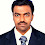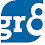# Partnership

Assume that TWO or MORE people together are doing a Business. It is called partnership. All the people who invests money are called Partners. The money contributed by these people is called their Capital (C).

Simple Partnership : If each person contributes only one capital for the same time period, then it is called a Simple Partnership. In a simple partnership the Profit / Loss is divided in the ratio of the Capitals.Compound Partnership : If the partners contribute different capitals for different capitals for different time periods or at least one partner contributes two or more capitals for different time periods then the partnership is called as the Compound Partnership. In Compound partnership the profit / loss will be divided in the ratio of Monthly Equivalent Investment (MEI).

MEI : MEI = Capital of a person X Respective investment period.

Sleeping Partner : If a person just invests the money but doesn't take care of day to day activities of the business is called a Sleeping Partner.

Active Partner : The person who actually takes care of the day to day activities of the business is called the Active Partner. The active partner will be paid a monthly salary or a part of gross profit at the end of the business term.

Important Note from Guide4BankExams : In every question that is being asked in bank exams whether the partnership is Simple or Compound, always take the ratio of Capitals including advances and withdrawals and then replace the Capitals with the respective terms of the Ratio.

Now lets do some problems.

1. Ranbir and Imran started a business initially with Rs. 14,200 and Rs. 15,600 respectively. If the total profit is Rs. 74,500 then what is Ranbir's share in the profit?

Sol : Capital's ratio of Ranbir and Imran = 14200 : 15,600  = 71:78
Their profit's ratio = 71:78

So, Ranbir's Profit =  ((74,500) / (71+78)) X 71 = (74500 / 149) x 71 = 35,500

2. A and B enter into a partnership by making investments in the ratio 1:2. 5% of the total profit goes to charity. If B's share is Rs. 760, then total profit is?

Sol : Let the profit be 100% . Profit to be distributed by A and B,
So100% - 5% = 95% (Because 5% goes to Charity)

So, Capitals are in the ratio 1:2. They must distribute the profits in the ratio of 1:2.

So, B's share = ((95% / (1+2) ) X 2 = 190% / 3

=> 190%/3  =  960

So 100%  ---->  ?

= (760 x 100%) / ( 190%/3) =  (760 x 300) / 190 = 1200

3. Amir, Sharukh and Salman started a business jointly investing Rs. 11 Lakh, Rs 16.5 Lakh and Rs 8.25 lakh respectively. The profit earned by them in the business at the end of 3 years was Rs. 19.5 Lakh. What will be the 50% of Salman's share in the profit?

Sol :  Capitals ratio of Amir, Sharukh and Salman = 11 : 16.5 : 8.25
= 44 : 66 : 33   (Just tripled the values and added to avoid DECIMALS)
= 4 : 6 : 3
Since all of them invest for the same time period ( 3 Years), their partnership is a Simple One.

So, profit's Ratio = Capital's Ratio = 4 : 6 : 3

Total profit = 19.5 Lakhs

So, Salman's Share = ( (19.5) / (4+6+3) ) X 3 = ( (19.5) / 13) ) X 3 = 4.5

So, 50% of  Salman's Share = 4.5 / 2 = 2.25 Lakhs

4. A, B, C and D enter into a partnership investing Rs. 3000, C invests twice as much as D. B invests twice as much as C and A invests twice as much as B. Find B's share of the annual profit of Rs 1250?

Sol :  Let D=1, C=2X1 = 2, B=2X2=4, A=2X4=8

=>  A:B:C:D = 8:4:2:1

So, B's Share in the profit of Rs. 2250 = ((2250) / (8+4+2+1)) X 4 = ((2250 / 15) X 4) = 600

5. A, B and C enter into a partnership with certain capital in which A's Contribution is Rs. 10,000. If out of total profit of Rs 1000. A gets Rs. 500. B gets Rs 300, then C's capitals is?

Sol: Profit's ratio of A:B:C

= 500 : 300 : 200 = 5 : 3 : 2

So, Capital's A : B : C = 5 : 3 : 2

So, C's Capital = (10000 / 5)  X 2 = 4000

6. A and B started a business by investing Rs 50,000 and Rs 60,000 respectively.  A receives 12 1/2 % of the profit for managing the business and the rest is divided in proportion to their capitals. Find the share of each in a profit of    Rs 8800?

Sol: Capital's ratio of A and B = 50000 : 60000 = 5:6

= 12 1/2 % X 8800 = (1/8) X 8800 = 1100

Profit to be distributed = 8800 - 1100 = 7700

So, B's Share = (7700 / (5+6)) X 6 =  (7700 / 11) X 6 = 4200

Read more Aptitude Shortcut Techniques from Here

1.can you explain 3rd some if we tripled the values 11 it becomes 33 how it can become 44.

1.3 rd sum just multiply the value by 4 each.. to avoid the decimal values.

2.can you explain 3rd sum how it can changed 11 into 44 if we tripled the values

1.Well, you might not got the concept. See, here we have decimals (16.5 and 8.25). We cant do calculations with these sort of numbers. So you just should remove these decimal values. YOu can do what ever you can to make them Rounded. Here we've just tripled the actual number and added to it... So that we could avoid decimals. Dont be confused.

Hope it clears your doubt now.

3.in sixth question how it becomes 1/8 from 12 1/2 ?

1.Its 12 1/2 % friend...

that means 12 1/2 * (1/100) (percent means you should multiply with 1/100)

that will become 25/2 * (1/100)

=> 25/200 => 1/8

hope you got it now :)

4.in 4th question how u get 2250?

5.6.question 3. annual profit is Rs-1250 instead od Rs-2250/-

7.Question-3 annual profit is -Rs-1250 instead od Rs-2250.

8.Answewr no-5 Total profit is Rs-1000. A get 500 B get 300 and balance Rs-200 for C . hence the the C share of Capital 10000/5*2=4000, becoz capital A's is given Rs-10000/-

1.how did you get 5?